Complex numbers

This free course is available to start right now. Review the full course description and key learning outcomes and create an account and enrol if you want a free statement of participation.

Free course

# 3.3 Section summary

In this section we have seen a correspondence between complex numbers and points in the plane using Cartesian coordinates; the real part of the complex number is represented on the real axis (“horizontal”) and the imaginary part on the imaginary axis (“vertical”). We can also use polar coordinates (r,θ) in which case r, the modulus of a non-zero complex number z is positive and θ is an argument of z, defined only to within an additive integer multiple of 2. The principal value of the argument is that value of θ in (−], and is denoted by Arg z.

Multiplication and division are particularly easy operations to carry out when the numbers are in polar form. We discussed the conjugate of a complex number and how to find the nth roots of a complex number.

## Example 3

(i) If z = i, then |z| = 1 and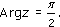(ii) If z = −1, then |z| = 1 and Arg z =.

(iii) If z =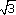i then |z| =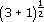= 2 and Arg = −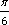It is usually a good idea to scribble a little diagram like Figure 11 to make sure that you get the correct angle for Arg z.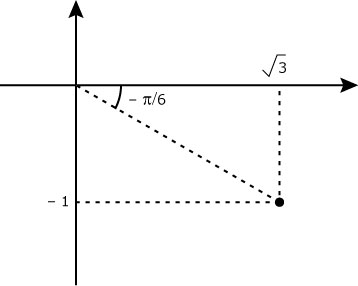Figure 11

(iv)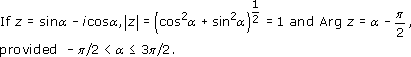In Figure 12 we have taken α(0,/2).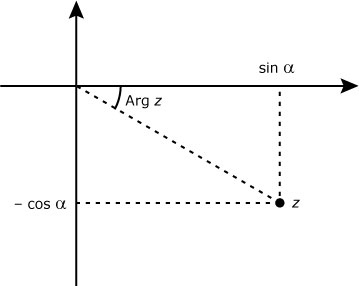Figure 12

## Example 4

(i)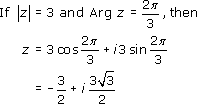(Figure 13).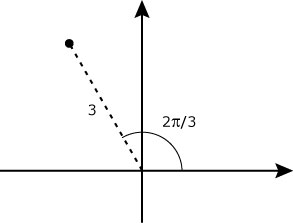Figure 13

(ii)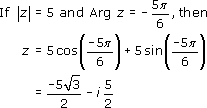(Figure 14).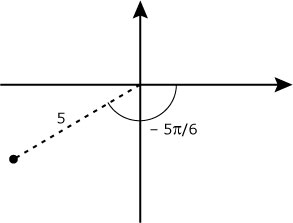Figure 14

## Example 5

(i)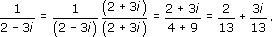(ii)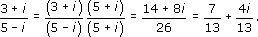## Example 6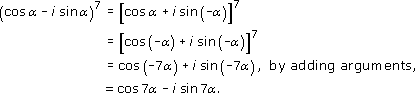M332_1

### Take your learning further

Making the decision to study can be a big step, which is why you'll want a trusted University. The Open University has 50 years’ experience delivering flexible learning and 170,000 students are studying with us right now. Take a look at all Open University courses.

If you are new to university level study, find out more about the types of qualifications we offer, including our entry level Access courses and Certificates.

Not ready for University study then browse over 900 free courses on OpenLearn and sign up to our newsletter to hear about new free courses as they are released.

Every year, thousands of students decide to study with The Open University. With over 120 qualifications, we’ve got the right course for you.

Request an Open University prospectus